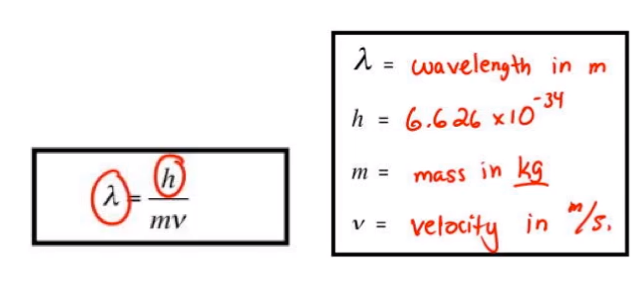# Problem: The resolution limit of a microscope is roughly equal to the wavelength of light used in producing the image. Electron microscopes use an electron beam (in place of photons) to produce much higher resolution images, about 0.23 nm in modern instruments. Assuming that the resolution of an electron microscope is equal to the de Broglie wavelength of the electrons used, to what speed must the electrons be accelerated to obtain a resolution of 0.23 nm?

###### FREE Expert Solution
• Use the de Broglie wavelength equation where• We need to find the v in m/s using the provided wavelength (0.23 nm) which is in nanometers (we need it in meters)
• Use the mass of electron which is 9.11x10-31 kg
96% (27 ratings)###### Problem Details

The resolution limit of a microscope is roughly equal to the wavelength of light used in producing the image. Electron microscopes use an electron beam (in place of photons) to produce much higher resolution images, about 0.23 nm in modern instruments. Assuming that the resolution of an electron microscope is equal to the de Broglie wavelength of the electrons used, to what speed must the electrons be accelerated to obtain a resolution of 0.23 nm?